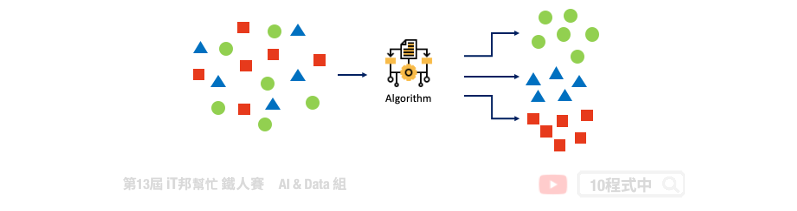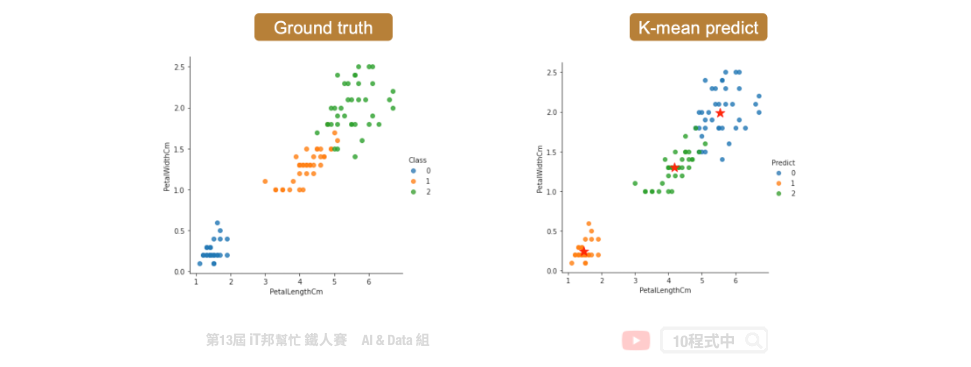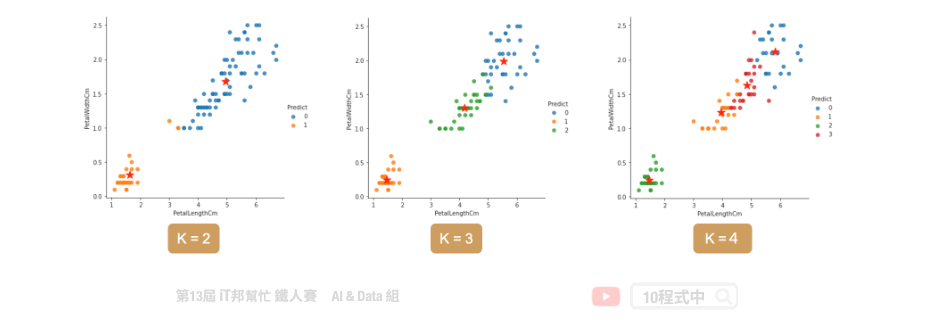DAY 6
1
AI & Data

# 非監督式學習 K-means 分群

## 今日學習目標

• 非監督式學習
• 何謂非監督式學習? 集群分析?
• 分群演算法介紹
• K-means 分群分類演算法

## 非監督式學習(Un-supervised learning)## K-means 演算法

1. 初始化: 指定 K 個分群，並隨機挑選 K 個資料點的值當作群組中心值
2. 分配資料點: 將每個資料點設為距離最近的中心
3. 計算平均值: 重新計算每個分群的中心點## 載入相關套件

``````import pandas as pd
import numpy as np
import matplotlib.pyplot as plt
import seaborn as sns
``````

## 1) 載入資料集

``````iris = load_iris()
df_data = pd.DataFrame(data= np.c_[iris['data'], iris['target']],
columns= ['SepalLengthCm','SepalWidthCm','PetalLengthCm','PetalWidthCm','Species'])
df_data
``````

## K-Means

K-means 演算法在 Sklearn 套件中已經幫我們封裝好了，使用者只要呼叫 API 即可將分群分類演算法快速實作。

Parameters:

• n_cluster: K的大小，也就是分群的類別數量。
• random_state: 亂數種子，設定常數能夠保證每次分群結果都一樣。
• n_init: 預設為10次隨機初始化，選擇效果最好的一種來作為模型。
• max_iter: 迭代次數，預設為300代。

Attributes:

• inertia_: inertia_：float，每個點到其他叢集的質心的距離之和。
• cluster_centers_： 特徵的中心點 `[n_clusters, n_features]`

Methods:

• fit: K個集群分類模型訓練。
• predict: 預測並回傳類別。
• fit_predict: 先呼叫fit()做集群分類，之後在呼叫predict()預測最終類別並回傳輸出。
• transform: 回傳的陣列每一行是每一個樣本到kmeans中各個中心點的L2(歐幾里得)距離。
• fit_transform: 先呼叫fit()再執行transform()。
``````from sklearn.cluster import KMeans

kmeansModel = KMeans(n_clusters=3, random_state=46)
clusters_pred = kmeansModel.fit_predict(X)
``````

## 評估分群結果

``````kmeansModel.inertia_
``````

``````78.94084142614602
``````

``````kmeansModel.cluster_centers_
``````

``````array([[5.9016129 , 2.7483871 , 4.39354839, 1.43387097],
[5.006     , 3.428     , 1.462     , 0.246     ],
[6.85      , 3.07368421, 5.74210526, 2.07105263]])
``````

## 分類結果## 如何決定K?

• Inertia 計算所有點到每群集中心距離的平方和。
• silhouette scores 側影函數驗證數據集群內一致性的方法。### 使用 inertia 做模型評估

``````# k = 1~9 做9次kmeans, 並將每次結果的inertia收集在一個list裡
kmeans_list = [KMeans(n_clusters=k, random_state=46).fit(X)
for k in range(1, 10)]
inertias = [model.inertia_ for model in kmeans_list]
``````### 使用 silhouette scores 做模型評估

``````from sklearn.metrics import silhouette_score
silhouette_scores = [silhouette_score(X, model.labels_)
for model in kmeans_list[1:]]
``````